top of pageSearch

# Linear Algebra for ML Part 1 | Data Representation

Updated: Jan 23

## Starting From Using Matrix and Vector for Data RepresentationTruth be told, the role of linear algebra in machine learning has been perplexing me, as mostly we learn these concepts (e.g. vector, matrix) in a math context while discarding their applications in machine learning. In fact, linear algebra has several foundational use cases in machine learning, including data representation, dimensionality reduction and vector embedding. Starting from introducing the basic concepts in linear algebra, this article build a elementary view of how these concepts can be applied to represent data in data science, such as solving linear equation system, linear regression and neural networks.

## Definition of Scalar, Vector, Matrix and Tensor

Firstly, let’s address the building blocks of linear algebra - scalar, vector, matrix and tensor.

• scalar: a single number

• vector: an one-dimensional array of numbers

• matrix: a two-dimensional array of numbers

• tensor: a multi-dimensional array of numbersWe can implement them using Numpy array np.array() in python.

```scalar = 1
vector = np.array([1,2])
matrix = np.array([[1,1],[2,2]])
tensor = np.array([[[1,1],[2,2]],
[[3,3],[4,4]]])```

Let’s look at the shape of the vector, matrix and tensor we generated above.

```print('vector shape:', vector.shape)
print('matrix shape:', matrix.shape)
print('tensor shape:', tensor.shape)```## Matrix and Vector Operations

### 1. Addition, Subtraction, Multiplication, Division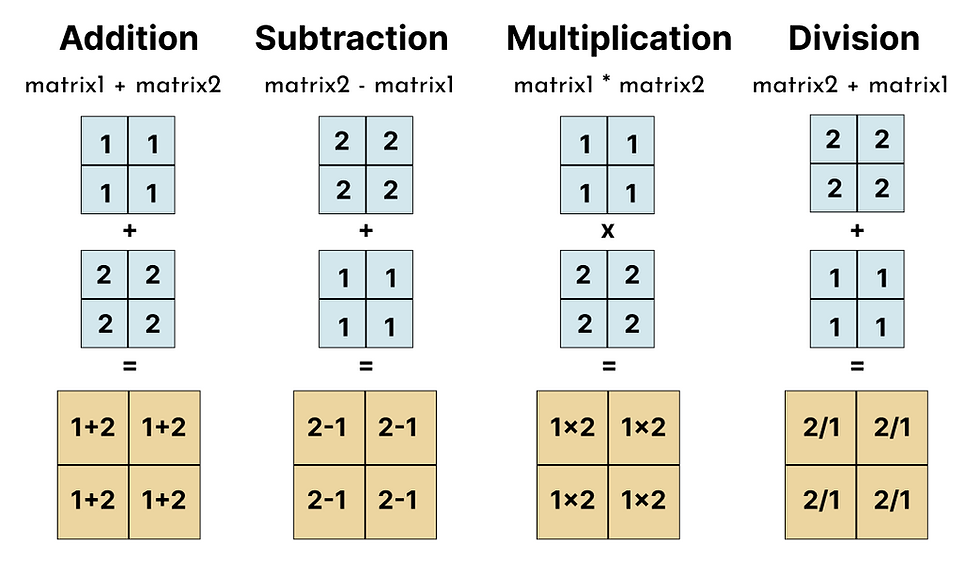Similar to how we perform operations on numbers, the same logic also works for matrices and vectors. However, please note that these operations on matrix have restrictions on two matrices being the same size. This is because they are operated in an element-wise manner, which is different from matrix dot product.### 2. Dot Product

Dot product is often being confused with matrix element-wise multiplication (which is demonstrated above), however, in fact it is a more commonly used operations on matrices and vectors.

Dot product operates by multiplying each row of the first matrix to the column of the second matrix, therefore the dot product between a j x k matrix and k x i matrix is a j x i matrix. Here is an example of the dot product between a 3x2 matrix and a 2x3 matrix.Dot product operation necessitates the number of columns in the first matrix matching the number of rows in the second matrix. We use dot() to execute the dot product. The order of the matrices in the dot product operations is crucial - matrix2.dot(matrix1) will produce a different result as shown below. Therefore, as opposed to element-wise multiplication, matrix dot product is not commutative.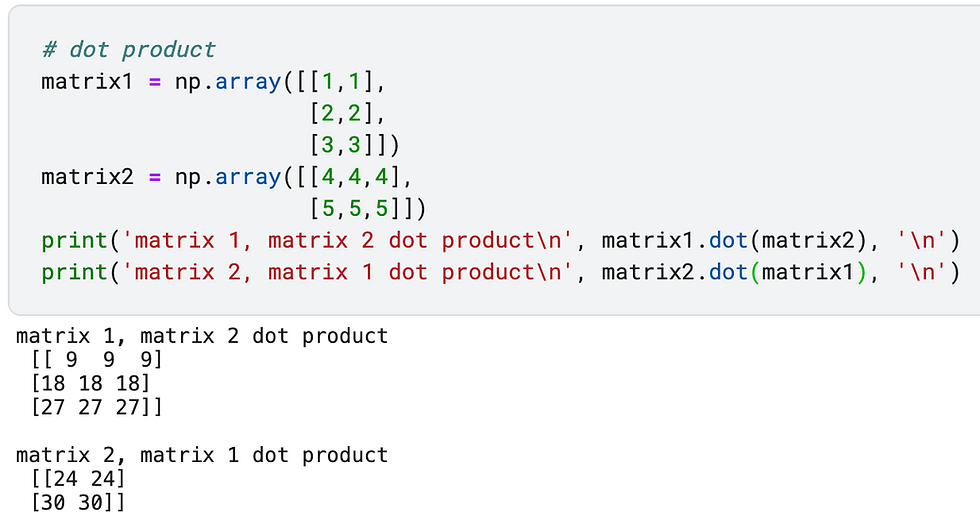### 3. ReshapeA vector is often seen as a matrix with one column and it can be reshaped into matrix by specifying the number of columns and rows using reshape(). We can also reshape the matrix into a different layout. For example, we can use the code below to transform the 2x2 matrix to 4 rows and 1 column.When the size of the matrix is unknown, reshape(-1) is also commonly used to reduce the matrix dimension and “flatten” the array into one row. Reshaping matrices can be widely applied in neural network in order to fit the data into the neural network architecture.### 4. TransposeTranspose swaps the rows and columns of the matrix, so that an j x k matrix becomes k x j. To transpose a matrix, we use matrix.T.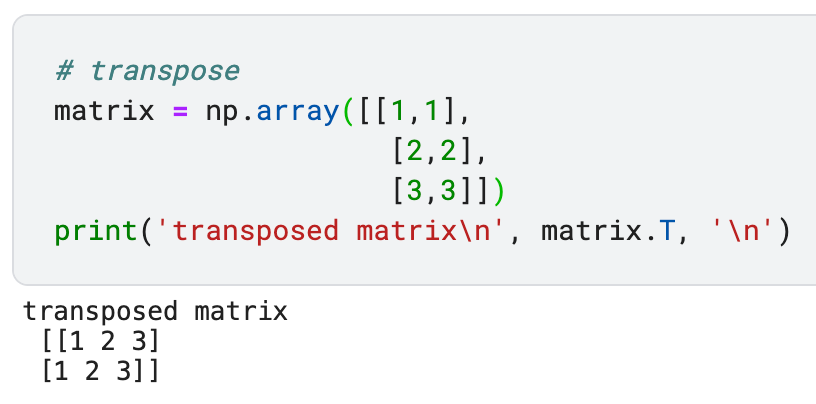### 5. Identity and Inverse MatrixInverse is an important transformation of matrices, but to understand inverse matrix we first need to address what is an identity matrix. An identity matrix requires the number of columns and rows to be the same and all the diagonal elements to be 1. Additionally, a matrix or vector remain the same after multiplying its corresponding identity matrix.

To create a 3 x 3 identity matrix in Python, we can use numpy.identity(3).The dot product of the matrix itself (stated as M below) and the inverse of the matrix is the identity matrix which follows the equationThere are two things to take into consideration with matrix inverse: 1) the order of the matrix and matrix inverse does not matter even though most matrix dot products are different when the order changes; 2) not all matrices have an inverse. To compute inverse of the matrix, we can use np.linalg.inv().If you would like to go deeper into these concepts, I found the book “Mathematics for Machine Learning” from Deisenroth, Faisal and Ong particularly helpful.

## Applications of Linear Algebra in ML

We will start with the most straightforward applications of vector and matrix in solving system of linear equations, and gradually generalize it to linear regression then neural networks.

### 1. Linear Algebra Application in Linear Equation System

Let us start with solving the system of linear equations using matrices. Suppose that we have the system below, a typical way to compute the value of a and b is to eliminate one element at a time.

3a + 2b = 7
a - b = -1

An alternative solution is to represent it using the dot product between matrix and vector. We can package all the coefficients into a matrix and all the variable into a vector, hence we get following:Let us represent the coefficient matrix as M, variable vector as x and output vector y, then multiply both side of the equation by inverse of the matrix M. Since the dot product between inverse of the matrix and the matrix itself is the identity matrix, we can simplify the linear equation system as solving the inverse of the coefficient matrix M and then taking the dot product of the output vector y.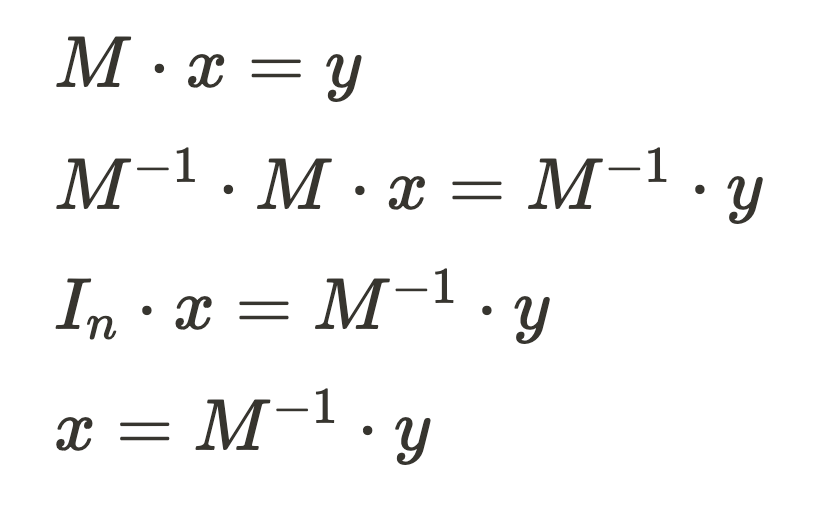We can use the code snippet below to compute the value of variable a and b.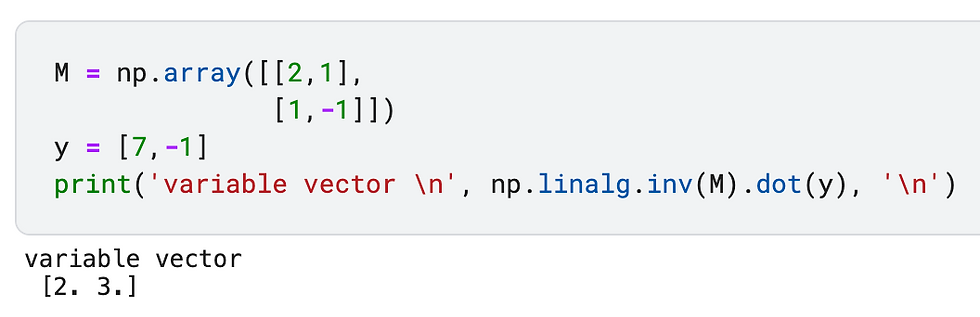By representing the linear equation systems using matrices, this increase the computational speed of the process significantly. Imagine that we are using the traditional method, it requires using several for-loops to eliminate one element at a time, however, using matrix method we can solve the [a,b] vector in one step. This may seem to be a small enhancement in such a simple system, but if we expand it to machine learning or even deep learning, it makes drastic increase in efficiency.

### 2. Linear Algebra Application in Linear Regression

The same principle shown in solving the linear equation system can be generalized to linear regression models in machine learning. If you would like to refresh your memory of linear regression, please check out my article on "A Simple and Practical Guide to Linear Regression".

Suppose that we have a dataset with n features and m instances, we typically represent linear regression as the weighted sum of these features.Let’s begin with representing an individual instance using the matrix form. We can store the feature values in a 1 x (n+1) matrix and the weights are stored in an (n+1) x 1 vector. Then we multiply the element with the same color and add them together to get the weighted sum.When the number of instances increase, we naturally think of using for loop to iterate an item at a time which can be time consuming. By representing the algorithm in the matrix format, the linear regression optimization process boils down to solving the coefficient vector [w0, w1, w2 … wn] through linear algebra operations.Additionally, popular Python libraries such as Numpy and Pandas build upon matrix representation and utilizes “vectorization” to speed up the data processing speed. I found the article “Say Goodbye to Loops in Python, and Welcome Vectorization!” quite helpful in terms of the comparison between the computation time of for-loop and vectorization.

### 3. Linear Algebra Application in Neural Network

Neural network is composed of multiple layers of interconnected nodes, where the outputs of nodes from the previous layers are weighted and then aggregated to form the input of the subsequent layers. If we zoom into the interconnected layers of a neural network, we can see some components of the regression model.Take a simple example that we visualize the inner process of the hidden layer i (with node i1, i2, i3) and hidden layer j (with node j1, j2) from a neural network. w11 represents the weight of the input node i1 that feeds into the node j1 and w21 represents the weight of input node i2 that feeds into node j1. In this case, we can package the weights into 3x2 matrix.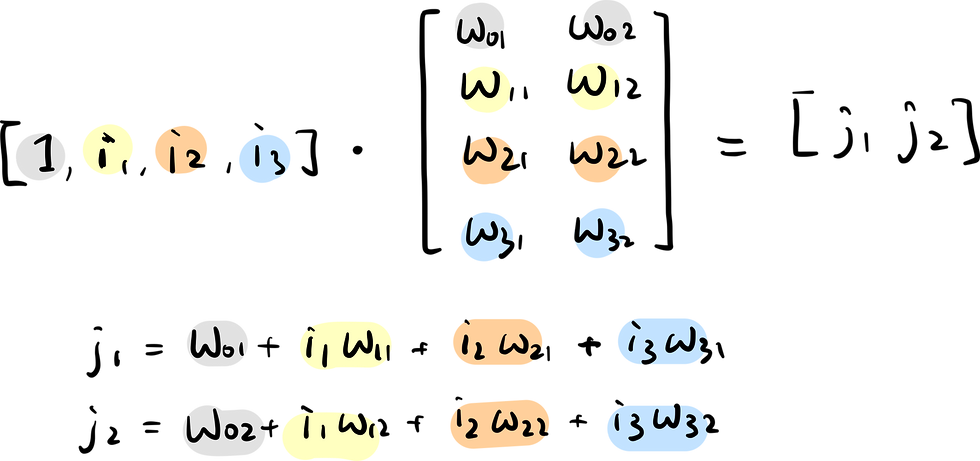This can be generalized to thousands or even millions of instances which forms the massive training dataset of neural network models. Now this process resembles how we represent the linear regression model, except that we we use a matrix to store the weights instead of a vector, but the principle remains the same.To take a step further, we can expand this to deep neural networks for deep learning. This is where Tensor come into play to represent data with more than two dimensions, for example, in Convolutional Neural Network image pixels are often depicted through three different channels (i.e., red, green, blue color channel).

## Take Home Message

The importance of linear algebra in machine learning may seem implicit, however, it plays a fundamental role in terms of data representation and more. This article we start with introducing basic concepts such as:

• scalar, vector, matrix, tensor

• addition, subtraction, multiplication, division, dot product

• reshape, transpose, inverse

Additionally, we discuss how these concepts have been applied in data science and machine learning, including

• linear equation system

• linear regression

• neural network

2,543 views1 comment
bottom of page